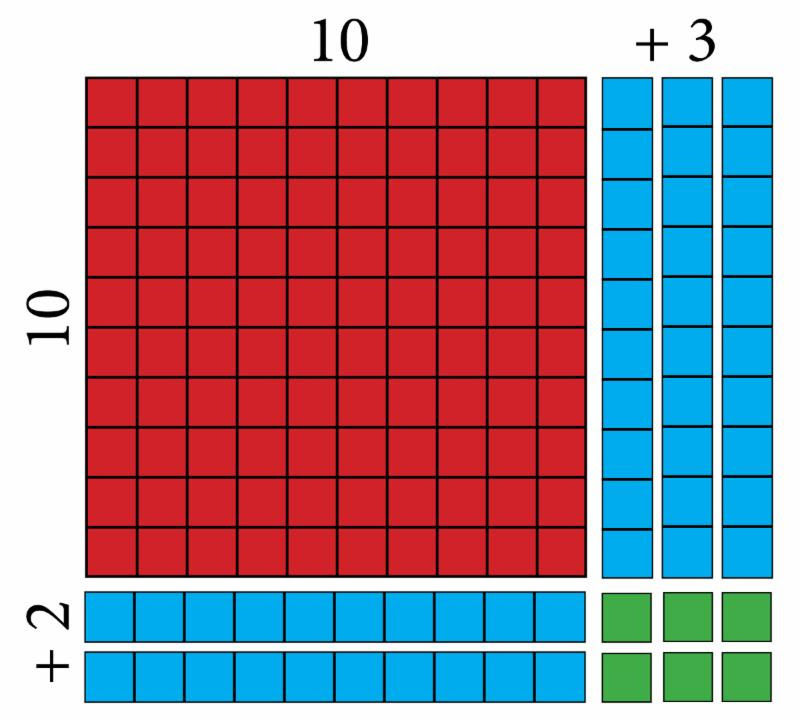top of page
Search

# 3 in 1: Making Math Meaningful

Updated: Nov 7, 2022

By David Quine

Combining principles of Schaeffer, Mason, and Moore

MAKING MATH MEANINGFUL Just how to combined the principles of Dr. Francis Schaeffer, Charlotte Mason, and Dr. Raymond Moore was the challenge. I first began this process in the writing of Making Math Meaningful in 1984. Dr. Francis Schaeffer THE PRINCIPLE: "Today we have a weakness in our educational process in failing to understand the natural associations between the disciplines. We tend to study all our disciplines in unrelated parallel lines...and the things of man are never unrelated parallel lines." THE PROCESS: When I was designing Making Math Meaningful, I realized that arithmetic, geometry, and algebra were an example of the problem Dr. Schaeffer was referring to. These three components of mathematics were all taught as "unrelated parallel lines" - that is, they were taught as separate "subjects" or separate chapters making the teaching and learning of math very fragmented. Therefore, I began looking for ways to unify the three parts into one whole. Whenever possible, I would teach arithmetic, geometry, and algebra at the same time - as one subject -  rather than three separate subjects. Charlotte Mason THE PRINCIPLE: "The chief value of arithmetic, like that of higher mathematics, lies in the training it affords to the reasoning powers." "A bag of beans, counters, or buttons should be used in all the early arithmetic lessons and the child should be able to work with these freely." THE PROCESS: Skills always follow concepts in Making Math MeaningfulEach new concept is first introduced with objects. In this way, students are always searching for patterns and relationships. All new concepts are introduced through the use of word problems. Because students first understand the concept and why it is necessary to learn the skill (in order to solve the problem), learning the skill is much easier. Dr. Raymond Moore THE PRINCIPLE: "...personal, informal, natural, and conversational." THE PROCESS: In the beginning I gave Shirley a math lesson plan for her to teach our children during the day while I was at work. At the end of the day when I came home, I asked her how it went. She said it didn't go well. What? How was that possible? What went wrong? Then she lovingly explained to me that what she was to do and say in order to make the lesson work was in "my head" but not hers. So from that day on I began writing lesson plans with two columns: On the left: "What I Am To Do" and on the right: "What I Am To Say". Making Math Meaningfulbecame an informal, natural conversation between Shirey and our children. Let me give you a specific example. The lesson plans for teaching double-digit multiplication begins with a conversation regarding a jigsaw puzzle: Bryce and Ben are working on a jigsaw puzzle. They want to know how many pieces there are altogether. The puzzle has 12 rows with 13 pieces in each row. How many pieces make up the puzzle? This problem involves: 1 - Arithmetic - multiplying 12 x 13, 2 - Geometry - finding the area of a rectangle, l x w, and  3 - Algebra - multiplying two binomials (x + 2)(x + 3) where x = 10 As you can see this is an example of "3 in 1" - three subjects in one problem. It also incorporates the ideas of Schaeffer, Mason, and Moore - "3 in 1." This approach is much more effective and efficient.Students replicate the puzzle using graph paper. Students are asked: 10 plus "how many ?" equals 12 10 plus "how many ?" equals 13 Using the graph paper squares, replicate the puzzle using one 10 x 10 square, five 1 x 10 strips, and six 1 x 1 squares. The process of teaching the skill 12 x 13 starts with "mental math" ... The 10 by 10 red square has how many small squares? (100) Three ten strips plus two ten strips is how many small squares? (50) And there are six small single squares. How many small squares (that is, puzzle pieces) are there in the complete puzzle? (100 + 30 + 20 + 6 = 156). The lesson plan continues teaching ... Arithmetic: 12 x 13 = 156 Geometry:length x wide = area, and Algebra: (x + 2)(x + 3) = (x)(x) + 5x + 6, where x = 10.

When teaching math, combining arithmetic, geometry, and algebra is the most  efficient way for students to learn by learning three "subjects" at one time. But more importantly, it is the most effective way for students to "own it" for themselves.

This is just one example of how we have combined the educational thoughts of Francis Schaeffer, Charlotte Mason, and Raymond Moore - "3 in 1" - into Cornerstone Curriculum's  Making Math Meaningful.

1 comment

See All
bottom of page